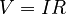# Voltage facts for kids

Kids Encyclopedia Facts
(Redirected from Electric voltage)

Voltage is a force that makes electricity move through a wire. It is measured in volts. Voltage is also called electric tension or electromotive force (EMF). It was named after Alessandro Volta.

Technically, the voltage is the difference in electric potential between two points. Voltage is always measured between two points, for example between the positive and negative ends of a battery, or between a wire and ground.

As seen in volt#Hydraulic analogy, voltage can be seen as the pressure on the electrons to move out of the source. It is directly proportional to the pressure exerted on the electrons. In other words, the higher the voltage, the higher the pressure. For example, a battery of 3 volts will exert pressure on the electrons twice as hard as a battery of 1.5 volts.

The voltage can push the electrons into a component, like a resistor, creating a current. Usually, the voltage and the current are related by a formula (see impedance).

Note that there must be both voltage and current to transfer power (energy). For example, a wire can have a high voltage on it, but unless it is connected, nothing will happen. Birds can land on high voltage lines such as 12kV and 16kV without dying, because the current does not flow through the bird.

There are two types of voltage, DC voltage and AC voltage. The DC voltage (direct current voltage) always has the same polarity (positive or negative), such as in a battery. The AC voltage (alternating current voltage) alternates between positive and negative. For example, the voltage from the wall socket changes polarity 60 times per second (in America). The DC is typically used for electronics and the AC for motors.

## Mathematical definition

Mathematically, the voltage is the amount of work needed to move a charge of 1 coulomb from one position to the other.$V = I R$

Where V=Voltage, I=Current, R=Total resistance.

## Ground voltage

Voltage is always measured between two points, and one of them is often called the "ground", or the zero volt (0V) point. In most AC electrical installation there is eventually a connection to the real ground through a water pipe or any convenient metallic conductor buried underground, using the whole planet Earth as a reference voltage. In DC circuits, the negative end of a battery is often called the "ground", even though there is no connection to the real ground. Lightning rods are normally "grounded" through a copper cable on the side of a building and connected to a spike planted into the ground.

## Measuring tools

Some of the tools for measuring the voltage are the voltmeter and the oscilloscope.

The voltmeter measures the voltage between two points and can be set to the DC mode or the AC mode. The voltmeter can measure the DC voltage of a battery for example (typically 1.5V or 9V), or the AC voltage from the power socket on the wall (typically 120V).

For more complex signals, an oscilloscope can be used the measured the DC and/or AC voltage, for example to measure the voltage across a speaker.

## Potential difference

The voltage, or potential difference from point a to point b is the amount of energy in joules (as a result of electric field) required to move 1 coulomb of positive charge from point a to point b. A negative voltage between points a and b is one in which 1 coulomb of energy is required to move a negative charge from point a to b. If there is a uniform electric field about a charged object, negatively charged objects will be pulled towards higher voltages, and positively charged objects will be pulled towards lower voltages. The potential difference/Voltage between two points is independent of the path taken to get from point a to b. Thus, the voltage from a to b + the voltage from b to c will always equal the voltage from a to c.

## Images for kidsVoltage Facts for Kids. Kiddle Encyclopedia.